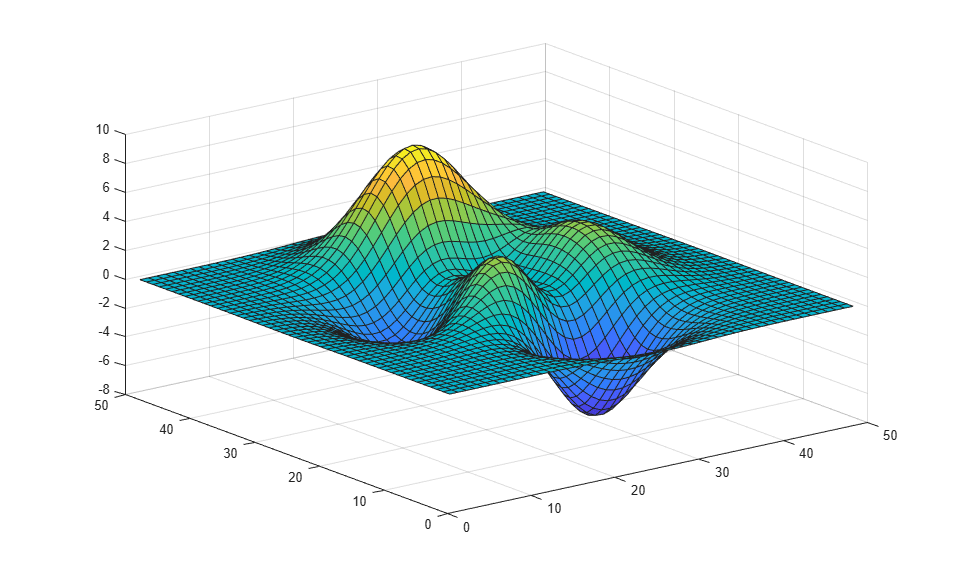# frame2im

Return image data associated with movie frame

## Syntax

``RGB = frame2im(F)``
``[X,map] = frame2im(F)``

## Description

example

````RGB = frame2im(F)` returns the truecolor (RGB) image from the single movie frame `F`.```
````[X,map] = frame2im(F)` returns the indexed image data `X` and associated colormap `map` from the single movie frame `F`.```

## Examples

collapse all

Create a surface plot. The data tip gives the x-, y- and z-coordinate of points along the surface.

```surf(peaks) ```Use `getframe` to capture the plot as a movie frame. The `'colormap'` field is empty, therefore the movie frame contains a truecolor (RGB) image.

```F = getframe ```
```F = struct with fields: cdata: [357x452x3 uint8] colormap: [] ```

Convert the captured movie frame to image data.

```RGB = frame2im(F); ```

Display the truecolor image. The data tip gives information about the column and row indices and RGB value of pixels.

```figure imshow(RGB) ```## Input Arguments

collapse all

Movie frame, specified as a structure with two fields:

• `cdata` — The image data stored as an array of `uint8` values.

• `colormap` — The colormap. If the movie frame contains a truecolor (RGB) images, then this field is empty (`[]`).

You can create a move frame structure by using the functions `im2frame` and `getframe`.

## Output Arguments

collapse all

Truecolor image, returned as an m-by-n-by-3 numeric array.

Data Types: `uint8`

Indexed image, returned as an m-by-n numeric matrix.

Data Types: `uint8`

Colormap associated with indexed image `X`, returned as a c-by-3 numeric matrix with values in the range [0, 1]. Each row of `map` is a three-element RGB triplet that specifies the red, green, and blue components of a single color of the colormap.

Data Types: `double`